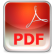Economic Themes (2008) 46 (2) 7, 79-93

## APPLICATION OF PATH ANALYSIS METHOD AND STRUCTURAL EQUATION MODELING IN MARKET RESEARCH

Vinko Lepojević, Vesna Janković-Milić

Abstract: Path analysis is a method which can be explained as an extention of regression analysis and can be used for testing of correlation matrix customizing toward two or more causality models compared from the reaserchers. Like an aspect of multiply regression which is focused on causality, path analysis can be considered as an separate case of structural equation modeling. Modeling of structural equation is a statistical method for validation and testing of causality relations using of statistical data combination and quality causal assumptions.

Keywords:  Path anaysis; modeling of structural equation; multiply regression; causation analysis